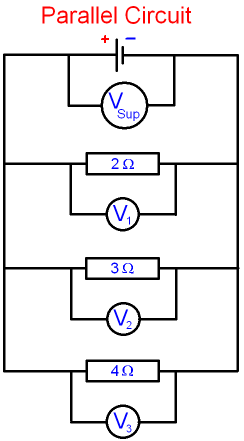gcsescience.com                                       23                                       gcsescience.com

Electricity

Parallel Circuits

What is the Voltage in a Parallel Circuit?

A parallel circuit is shown below with three
branches and a different resistor in each branch.

The cell (the power supply) and each resistor
has a voltmeter connected across it.It is found that

1. The voltage in a parallel circuit
is the same for all branches.  V1 = V2 = V3.

2. The voltage for each branch is the
same as the supply voltage.  V1 = V2 = V3= Vsup.

Each component behaves
as if it is connected independently to the cell.

gcsescience.com         Physics Quiz         Index         Electricity Quiz         gcsescience.com